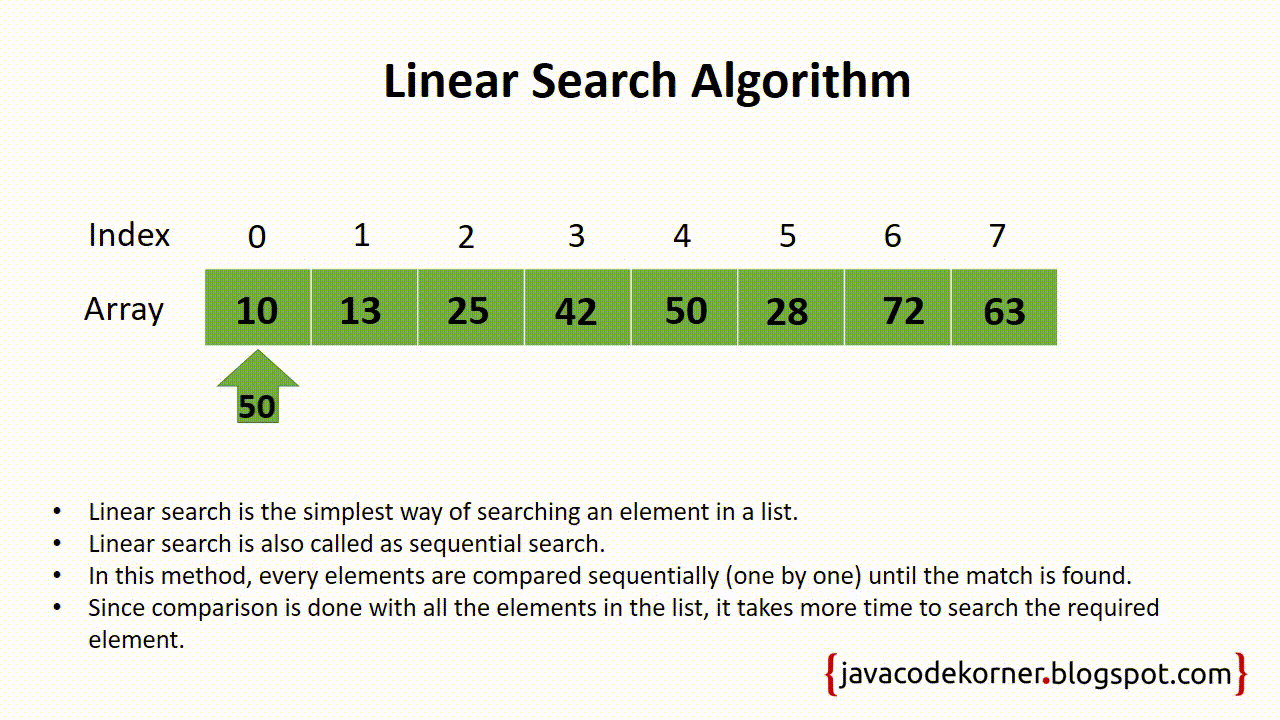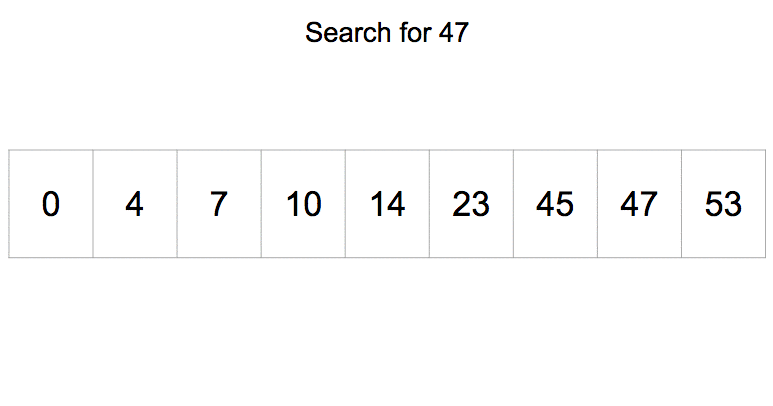# DSA — Binary Search Algorithm

A few months back ago I was interviewing for the position of Software Engineer in a reputed MNC. The day before the interview I concentrated on the important topics required for the interviews through multiple websites. However, what I failed to understand was that the most basic and most fundamental pillar is not the coding itself, it is

• How does the code and functions work?
• Which Algorithm is optimal to use?
• What are Data Structures and how to use them efficiently.

Unfortunately, I was rejected due to failure to attempt multiple questions from DSA. That was the day when I decided to explore more about DSA and at the same time, I thought of sharing it with others which would, in turn, help me learn too.

The first Most basic Algorithm which is quite famous and is used widely is Binary Search Algorithm.

# What is Binary Search Algorithm?

The main purpose of Binary Search Algorithm is to Search a Sorted Array to find a specific element. It tackles the problem of Linear Search Algorithm which is the time complexity. I will write a post about it sometime later.

# Short Description of Linear Search Algorithm

To give a short description of Linear Search Algorithm It will search the element one after the other. The representation for Linear Search Algorithm is:Linear Search Algorithm

# Steps for Binary Search Algorithm

For readability purpose I am considering the Index as Position.

Consider we want to find the position of the element 54 in the array [12, 13, 15, 17, 26, 37, 45, 54, 64, 75]. In Binary Search Algorithm, the steps that follow are:

1. It will first calculate and find the middle element of the array. It can be calculated by using the formula 0+n / 2 where n is the last element of the array starting from 0. In our case n = 9 (10 elements starting from 0) so the middle position would be 0+9/2 = 4.
2. Once the middle position is calculated (Position 4) , we will get the element on the middle position that is the 4th position which is 26 in our array.
3. When we get the element in the middle position (element = 26), we will check if the number which we desire to find (54) is either greater than or less than the element or equal to the element located at the middle of the array.(54 >26 OR 54 < 26 OR 54==26)
4. If it is equal to the middle element we will get the position of the element.
5. If it is greater than the middle element we will select the right array;
6. If it is less than the middle element we will select the left array
7. Since 54 > 26 we will select the right side of the array. So the new array now would be [37, 45, 54, 64, 75] and repeat step 1,2,3,4,5,6.

So if we look at it, Binary search just keeps dividing the array into 2 parts till the element that is to be searched is at the centre. The representation of the binary search algorithm is:Binary Search Algorithm

Binary Search Follows Decrease and Conquer Paradigm. The advantage of Binary search is that it is currently one of the most optimal solution to search a index(position) of the element. It has its drawbacks too, which is that the list or the array needs to be sorted for it to work.

The Representation of how binary search works vs how linear search works is:

So if you can see on the gif above. The target element 9 is found within 3 steps in Binary Search and is found in 9 steps in Linear Search Algorithm. Hence it increases the speed for larger data whereas the linear search speed decreases.

That’s all for now folks. This was my first article and hence would request you to spare a minute more to like, share and comment below with your honest and brutal feedback which will help me further improve my storytelling and my articles.

Hi! I am working as Sr. Software Engineer in IOT Sector. I have 5+ yrs experience in Python, node, IOT, C, C++ & Java. Lets catch up to know each other!

## More from Yatin Pandit

Hi! I am working as Sr. Software Engineer in IOT Sector. I have 5+ yrs experience in Python, node, IOT, C, C++ & Java. Lets catch up to know each other!